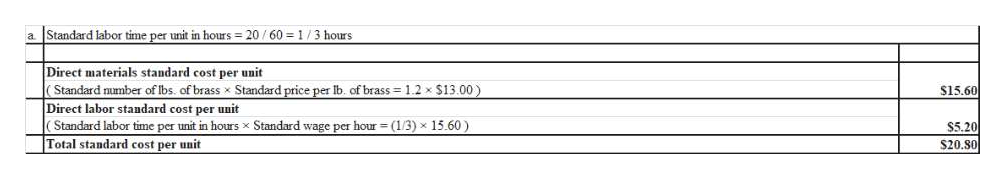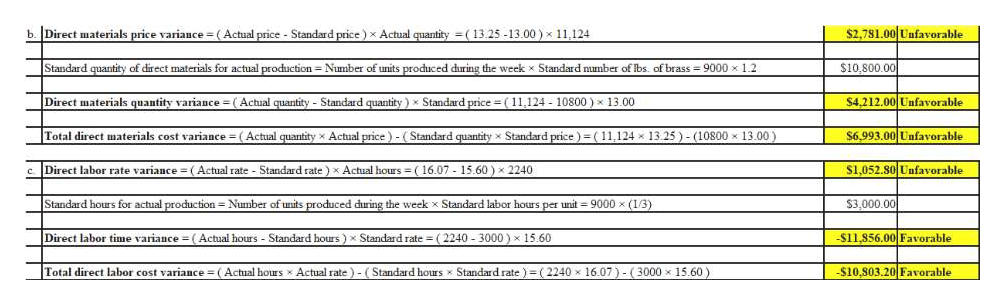# Direct Materials and Direct Labor Variance AnalysisShasta Fixture Company manufactures faucets in a small manufacturing facility. The faucets are made from brass. Manufacturing has 70 employees. Each employee presently provides 32 hours of labor per week. Information about a production week is as follows:Standard wage per hour\$15.60Standard labor time per unit20 min.Standard number of lbs. of brass1.2 lbs.Standard price per lb. of brass\$13.00Actual price per lb. of brass\$13.25Actual lbs. of brass used during the week11,124 lbs.Number of units produced during the week9,000Actual wage per hour\$16.07Actual hours for the week (70 employees × 32 hours)2,240Required:a. Determine the standard cost per unit for direct materials and direct labor. Round the cost per unit to two decimal places.Direct materials standard cost per unit\$Direct labor standard cost per unit\$Total standard cost per unit\$b. Determine the direct materials price variance, direct materials quantity variance, and total direct materials cost variance. Round your answers to the nearest whole dollar. Enter a favorable variance as a negative number using a minus sign and an unfavorable variance as a positive number.Direct Materials Price Variance\$ Direct Materials Quantity Variance\$ Total Direct Materials Cost Variance\$ c. Determine the direct labor rate variance, direct labor time variance, and total direct labor cost variance. Round your answers to the nearest whole dollar. Enter a favorable variance as a negative number using a minus sign and an unfavorable variance as a positive number.Direct Labor Rate Variance\$ Direct Labor Time Variance\$ Total Direct Labor Cost Variance\$

Question
53 views

Direct Materials and Direct Labor Variance Analysis

Shasta Fixture Company manufactures faucets in a small manufacturing facility. The faucets are made from brass. Manufacturing has 70 employees. Each employee presently provides 32 hours of labor per week. Information about a production week is as follows:

 Standard wage per hour \$15.60 Standard labor time per unit 20 min. Standard number of lbs. of brass 1.2 lbs. Standard price per lb. of brass \$13.00 Actual price per lb. of brass \$13.25 Actual lbs. of brass used during the week 11,124 lbs. Number of units produced during the week 9,000 Actual wage per hour \$16.07 Actual hours for the week (70 employees × 32 hours) 2,240

Required:

a. Determine the standard cost per unit for direct materials and direct labor. Round the cost per unit to two decimal places.

 Direct materials standard cost per unit \$ Direct labor standard cost per unit \$ Total standard cost per unit \$

b. Determine the direct materials price variance, direct materials quantity variance, and total direct materials cost variance. Round your answers to the nearest whole dollar. Enter a favorable variance as a negative number using a minus sign and an unfavorable variance as a positive number.

 Direct Materials Price Variance \$ Direct Materials Quantity Variance \$ Total Direct Materials Cost Variance \$

c. Determine the direct labor rate variance, direct labor time variance, and total direct labor cost variance. Round your answers to the nearest whole dollar. Enter a favorable variance as a negative number using a minus sign and an unfavorable variance as a positive number.

 Direct Labor Rate Variance \$ Direct Labor Time Variance \$ Total Direct Labor Cost Variance \$
check_circle

Step 1help_outlineImage Transcriptionclosea Standard labor time per unit in hours 20 /60 1/3 hours Direct materials standard cost per unit Standard number of Ibs. of brass x Standard price per Ib. of brass 1.2 x \$13.00) Direct labor standard cost per unit Standard labor time per unit in hours x Standard wage per hour (1/3) x 15.60) Total standard cost per unit \$15.60 \$5.20 \$20.80 fullscreen
Step 2help_outlineImage Transcriptionclose\$2,781.00 Unfavorable b. Direct materials price variance (Actual price Standard price) x Actual quantity (13.25-13.00) x 11,124 Standard quantity of direct materials for actual production Number of units produced during the week x Standard mumber of Ibs. of brass 9000 x 1.2 \$10,800.00 \$4.212.00 Unfavorable Direct materials quantity variance (Actual quantity Standard quantity) x Standard price (11,124 10800) x 13.00 Total direct materials cost variance (Actual quantity x Actual price) (Standard quantity x Standard price) ( 11,124 x 13.25)-(10800 x 13.00) \$6,993.00 Unfavorable s1,052.80 Unfavorable Direct labor rate variance (Actual rate Standard rate) x Actual hours (16.07 15.60) x 2240 Standard hours for actual production Number of units produced during the week x Standard labor hours per unit 9000 x (1/3) \$3,000.00 \$11,856.00 Favorable Direct labor time variance (Actual hours Standard hours) x Standard rate (2240 3000) x 15.60 \$10,803.20 Favorable Total direct labor cost variance (Actual hours x Actual rate) (Standard hours x Standard rate) - (2240 x 16.07)-(3000 x 15.60) fullscreen

### Want to see the full answer?

See Solution

#### Want to see this answer and more?

Solutions are written by subject experts who are available 24/7. Questions are typically answered within 1 hour.*

See Solution
*Response times may vary by subject and question.
Tagged in